Courses

# Study Notes for Sequential Circuits (Part -1) Electrical Engineering (EE) Notes | EduRev

## Electrical Engineering (EE) : Study Notes for Sequential Circuits (Part -1) Electrical Engineering (EE) Notes | EduRev

The document Study Notes for Sequential Circuits (Part -1) Electrical Engineering (EE) Notes | EduRev is a part of Electrical Engineering (EE) category.
All you need of Electrical Engineering (EE) at this link: Electrical Engineering (EE)

1. Introduction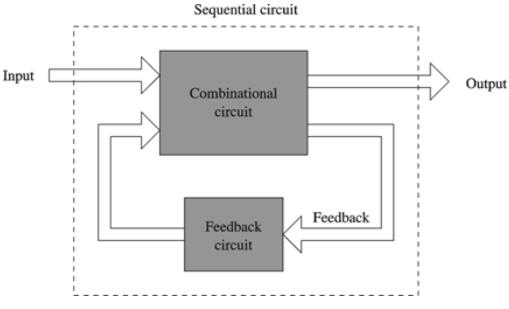The sequential circuit is of two types.

• Synchronous Sequential Circuit: Change in input signals can affect memory elements only upon activation of clock signals.
• Asynchronous Sequential Circuit: Change in input signals can affect memory elements at any instant of time. These are faster than the synchronous circuit.

2. Flip Flops

• It is a one-bit memory cell which stores the 1-bit logical data (logic 0 or logic 1).
• It is a basic memory element.
• The most commonly used application of flip flops is in the implementation of a feedback circuit.
• As a memory relies on the feedback concept, flip flops can be used to design it.
• In the synchronous sequential circuit, Memory elements are clocked flip flops and generally edge triggered.
• In the asynchronous sequential circuit, Memory elements are unclocked flip flops/time delay elements which are generally level triggered.
• Flip flop circuit is also known as bistable multivibrator or latch because it has two stable states (1 state, 0 states).

There are mainly four types of flip flops that are used in electronic circuits.

• S-R Flip Flop (Basic Flip Flop)
• Delay Flip Flop (D Flip Flop)
• J-K Flip Flop
• T Flip Flop

Basic SR Flip Flop

• The Set-Reset (SR) flip flop is designed with the help of two NOR gates or two NAND gates.
• SR Flip Flop is also called as SR latch.

SR Latch Implementation Using NAND Gates: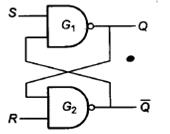Logic diagram of SR latch using NAND gates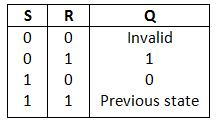Truth Table of Logic Diagram

SR Latch Using NOR Gates: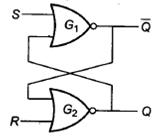Logic diagram of SR latch using NOR gates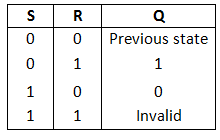Truth Table of Logic Diagram

Clocked SR Flip Flop Implementation using NAND Gates:
It is also called a Gated S-R flip flop. The problem with S-R flip flops using NOR and NAND gate is the invalid state. This problem can be overcome by using a bistable SR flip-flop that can change outputs when certain invalid states are met, regardless of the condition of either the Set or the Reset inputs.

• SR Flip Flop Using NOR Gates: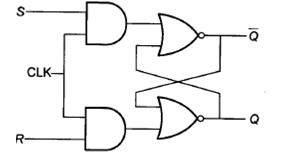Logic diagram of SR flip flop using NOR gates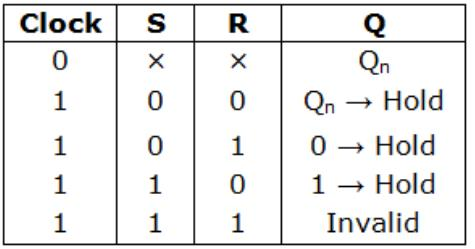Truth Table of SR Flip Flop
With both S=1 and R=1, the occurrence of a clock pulse causes both outputs to momentarily go to 0. When the pulse is removed, the state of the flip-flop is indeterminate, depending on whether the set or reset input of the flip-flop remains a 1 longer than the transition to 0 at the end of the pulse.
Characteristic Table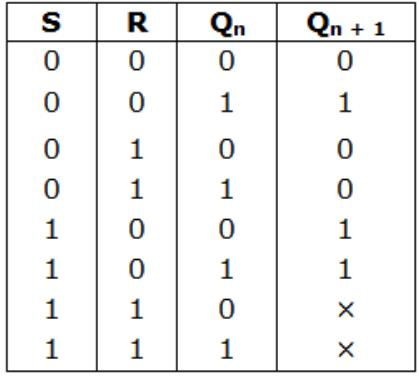Characteristic equation of SR flip flop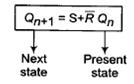Excitation Table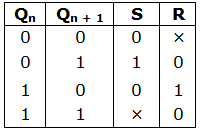JK Flip Flop
A JK flip-flop eliminates the indeterminate state of the SR type. Inputs J and K behave like inputs S and R to set and clear the flip-flop (In JK flip-flop, the letter J is set and the letter K is for clear). When logic 1 inputs are applied to both J and K simultaneously, the flip-flop switches to its complement state. If Q = 1, it switches to Q = 0 and vice versa.

• JK flip flop using SR flip flop:
S = JQ'
R = KQ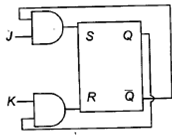• JK flip flop using NAND latch: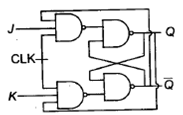• JK flip flop using NOR latch: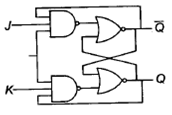Characteristic Table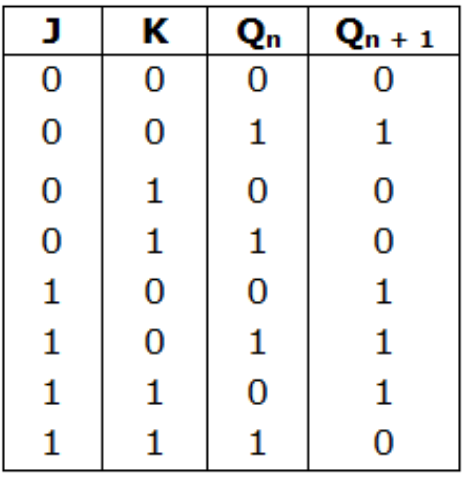Excitation Table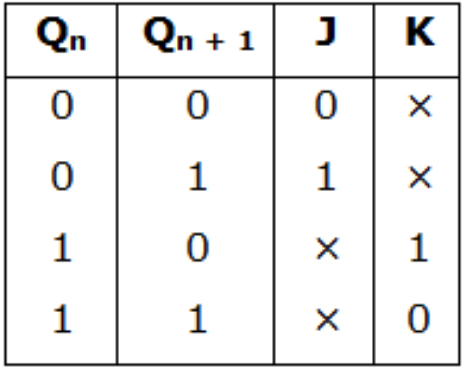• Characteristic equation for JK flip flop: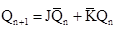D-Flip Flop
D flip flop is also known as a Transparent latch, Delay flip flop or data flip flop. The D input goes directly into the S (J) input and the complement of the D input goes to the R (K) input.

• The D-flipflop has only a single data input (D).
• If D = 1, the flip-flop is switched to the set state (unless it was already set).
• If D = 0, the flip-flop switches to the clear state.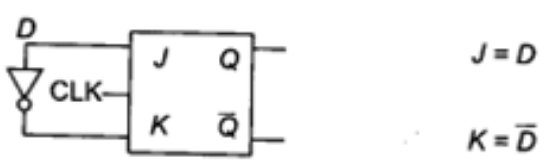Truth Table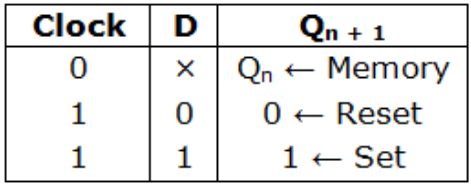Characteristic Table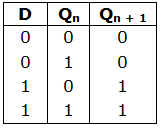Excitation Table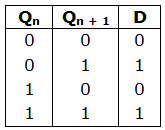• Characteristic equation for D-flop flop
Qn + 1 = D

T – Flip Flop

• The T flip-flop is a single input version of the JK flip-flop where T is connected to both J and K inputs directly.
• When T = 0, the flip flop enters into Hold mode, which means that the output, Q is kept the same as it was before the clock edge.
• When T = 1, the flip flop enters into Toggle mode, which means the output Q is negated after the clock edge, compared to the value before the clock edge.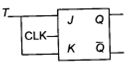Truth Table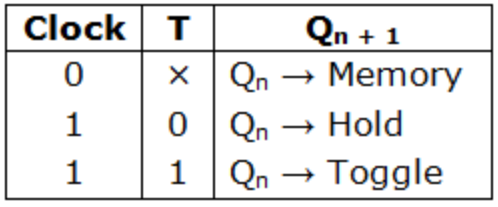Characteristic Table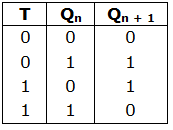Excitation Table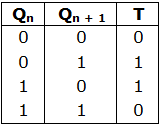• The characteristic equation of T-Flip Flop:
Qn + 1 = T ⊕ Qn
• Race Around Condition:
The race around condition will occur in JK flip flop when J = K = 1 and tpd (FF) < tpw.
To avoid race around condition.
tpw < tpd (FF) < TCLK

3. Master Slave (MS) Flip Flop

• A master-slave flip-flop is constructed from two separate flip-flops. One circuit serves as a master and the other as a slave. Input clock is applied to master and Inverted clock applied to slave.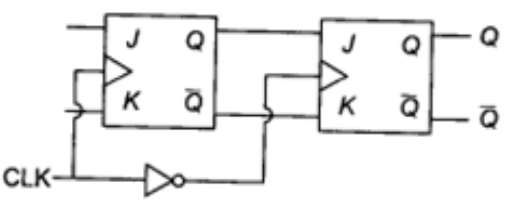• In Master Slave, flip flop output is changed only when slave output is changing.
• The master flip-flop is enabled on the positive edge of the clock pulse and the slave flip-flop is disabled by the inverter.
• The information at the external J and K inputs is transmitted to the master flip-flop.
• When the pulse returns to 0, the master flip-flop is disabled and the slave flip-flop is enabled. The slave flip-flop then goes to the same state as the master flip-flop.
• Master is level triggered, and Slave is edge triggered
• No race around condition occurs in Master Slave flip flop.
• It stores only one bit.

4. Flip Flop Conversions
The flip flop conversions are classified into different types which are:

• SR-FF to JK-FF Conversion
• JK-FF to SR-FF Conversion
• SR-FF to D-FF Conversion
• D-FF to SR-FF Conversion
• JK-FF to T-FF Conversion
• JK-FF to D-FF Conversion
• D-FF to JK-FF Conversion

Procedure for Flip Flop conversion:

1. Conversion Table: Construct the characteristic table of required flip flop (unknown), and fill available (known) flip flop excitation.
2. Solve K map for given (known) flip flop as input and required flip flop as output.
3. Implement the required flip flop using the known flip flop.

Example: Conversion from JK flip flop to D flip flop is shown below.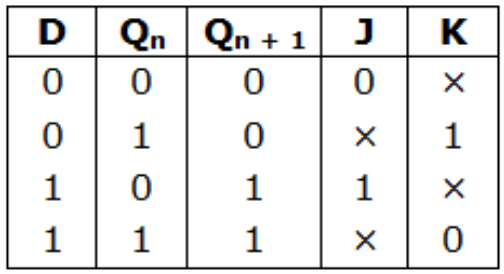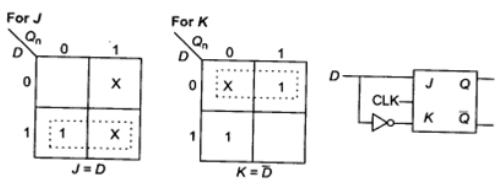Characteristic Table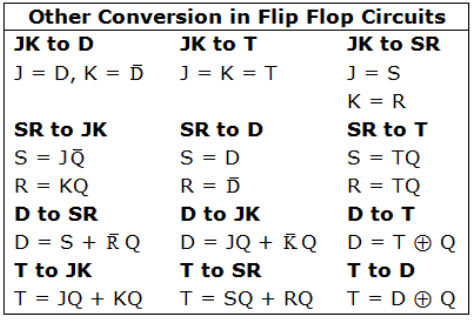Offer running on EduRev: Apply code STAYHOME200 to get INR 200 off on our premium plan EduRev Infinity!

,

,

,

,

,

,

,

,

,

,

,

,

,

,

,

,

,

,

,

,

,

;